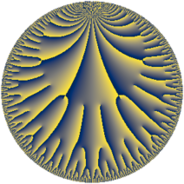# Properties

 Label 25.22.aLevel $25$ Weight $22$ Character orbit 25.a Rep. character $\chi_{25}(1,\cdot)$ Character field $\Q$ Dimension $32$ Newform subspaces $6$ Sturm bound $55$ Trace bound $2$

# Related objects

## Defining parameters

 Level: $$N$$ $$=$$ $$25 = 5^{2}$$ Weight: $$k$$ $$=$$ $$22$$ Character orbit: $$[\chi]$$ $$=$$ 25.a (trivial) Character field: $$\Q$$ Newform subspaces: $$6$$ Sturm bound: $$55$$ Trace bound: $$2$$ Distinguishing $$T_p$$: $$2$$

## Dimensions

The following table gives the dimensions of various subspaces of $$M_{22}(\Gamma_0(25))$$.

Total New Old
Modular forms 55 35 20
Cusp forms 49 32 17
Eisenstein series 6 3 3

The following table gives the dimensions of the cuspidal new subspaces with specified eigenvalues for the Atkin-Lehner operators and the Fricke involution.

$$5$$Dim.
$$+$$$$15$$
$$-$$$$17$$

## Trace form

 $$32 q - 1310 q^{2} - 6590 q^{3} + 32915734 q^{4} + 32120394 q^{6} - 428951550 q^{7} - 7470710280 q^{8} + 80388051186 q^{9} + O(q^{10})$$ $$32 q - 1310 q^{2} - 6590 q^{3} + 32915734 q^{4} + 32120394 q^{6} - 428951550 q^{7} - 7470710280 q^{8} + 80388051186 q^{9} + 58763249334 q^{11} + 243789580880 q^{12} + 23264691220 q^{13} - 506951114052 q^{14} + 32112566276562 q^{16} - 3785812896680 q^{17} - 79878729601190 q^{18} - 24154696946670 q^{19} + 182223686292744 q^{21} + 175723908487480 q^{22} - 314700067836570 q^{23} - 67037684537010 q^{24} + 2014979553112524 q^{26} + 1055133403919020 q^{27} - 1299171721121280 q^{28} + 4820308939119420 q^{29} - 5585445646540376 q^{31} - 2420800928707360 q^{32} + 35220308652025520 q^{33} - 8284606831073002 q^{34} + 6980250692091432 q^{36} - 49064421428406340 q^{37} - 40457968300985720 q^{38} + 127347070012212372 q^{39} + 261437066428652094 q^{41} + 362988657678789120 q^{42} - 201505254636709550 q^{43} - 179301115045948542 q^{44} - 60161303411641196 q^{46} - 194004714502145870 q^{47} + 308249523197103680 q^{48} + 1270188082523538524 q^{49} + 1415488094879008494 q^{51} - 1873978492365746200 q^{52} + 494105394664988260 q^{53} + 2792566678962993630 q^{54} - 1110996081424157220 q^{56} - 7087781283410679560 q^{57} + 12873215227165950620 q^{58} + 7953250308224170440 q^{59} - 11953639026389589116 q^{61} - 27985731899308528320 q^{62} - 4251178160982943710 q^{63} + 35523556004777871234 q^{64} + 34000825488469728378 q^{66} - 27434750902485096330 q^{67} - 2901526404081532840 q^{68} + 57893355838380787512 q^{69} + 3521670663441710604 q^{71} - 280723812246152312040 q^{72} + 77387927225337815080 q^{73} + 271638942795379469448 q^{74} - 156453492294789262990 q^{76} - 465454454501233790400 q^{77} + 455904321870956375600 q^{78} + 476055374305712350020 q^{79} + 93560094364982400732 q^{81} - 793438364204538301420 q^{82} + 275334409479725858490 q^{83} + 1513753550869256027028 q^{84} + 416866803375602317464 q^{86} - 1559745135897410874740 q^{87} + 736206990879619028640 q^{88} + 2404945959225835938510 q^{89} + 928037267440372207324 q^{91} - 3607084121648970468480 q^{92} + 675459022106427394920 q^{93} + 2386779789781437900848 q^{94} + 1191732516861701720574 q^{96} - 1991400758443910752720 q^{97} + 1036011348253477262930 q^{98} + 4090869190521055886832 q^{99} + O(q^{100})$$

## Decomposition of $$S_{22}^{\mathrm{new}}(\Gamma_0(25))$$ into newform subspaces

Label Dim $A$ Field CM Traces A-L signs $q$-expansion
$a_{2}$ $a_{3}$ $a_{5}$ $a_{7}$ 5
25.22.a.a $1$ $69.869$ $$\Q$$ None $$288$$ $$128844$$ $$0$$ $$768078808$$ $+$ $$q+288q^{2}+128844q^{3}-2014208q^{4}+\cdots$$
25.22.a.b $3$ $69.869$ $$\mathbb{Q}[x]/(x^{3} - \cdots)$$ None $$1312$$ $$-52194$$ $$0$$ $$-684416558$$ $+$ $$q+(437-\beta _{1})q^{2}+(-17400-8\beta _{1}+\cdots)q^{3}+\cdots$$
25.22.a.c $4$ $69.869$ $$\mathbb{Q}[x]/(x^{4} - \cdots)$$ None $$-2910$$ $$-83240$$ $$0$$ $$-512613800$$ $+$ $$q+(-728+\beta _{1})q^{2}+(-20803-14\beta _{1}+\cdots)q^{3}+\cdots$$
25.22.a.d $7$ $69.869$ $$\mathbb{Q}[x]/(x^{7} - \cdots)$$ None $$-737$$ $$-50381$$ $$0$$ $$-817782442$$ $+$ $$q+(-105-\beta _{1})q^{2}+(-7198+2\beta _{1}+\cdots)q^{3}+\cdots$$
25.22.a.e $7$ $69.869$ $$\mathbb{Q}[x]/(x^{7} - \cdots)$$ None $$737$$ $$50381$$ $$0$$ $$817782442$$ $-$ $$q+(105+\beta _{1})q^{2}+(7198-2\beta _{1}+\beta _{2}+\cdots)q^{3}+\cdots$$
25.22.a.f $10$ $69.869$ $$\mathbb{Q}[x]/(x^{10} - \cdots)$$ None $$0$$ $$0$$ $$0$$ $$0$$ $-$ $$q+\beta _{1}q^{2}+(6\beta _{1}-\beta _{4})q^{3}+(927372+\cdots)q^{4}+\cdots$$

## Decomposition of $$S_{22}^{\mathrm{old}}(\Gamma_0(25))$$ into lower level spaces

$$S_{22}^{\mathrm{old}}(\Gamma_0(25)) \cong$$ $$S_{22}^{\mathrm{new}}(\Gamma_0(1))$$$$^{\oplus 3}$$$$\oplus$$$$S_{22}^{\mathrm{new}}(\Gamma_0(5))$$$$^{\oplus 2}$$